1. /
2. CBSE
3. /
4. Class 06
5. /
6. Mathematics
7. /
8. NCERT Solutions for Class...

# NCERT Solutions for Class 6 Maths Exercise 7.1

NCERT solutions for Maths Fractions## NCERT Solutions for Class 6 Maths Fractions

###### Question 1.Write the fraction representing the shaded portion: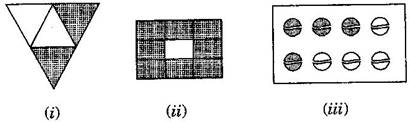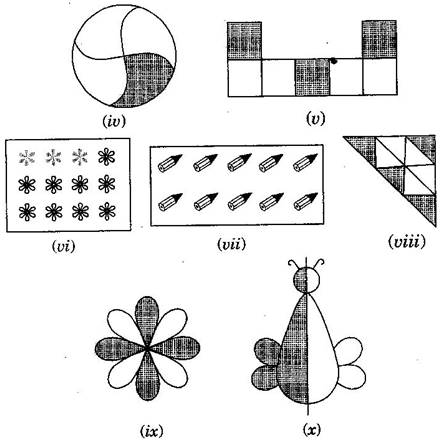(i){tex}\frac{2}{4}{/tex}

(ii){tex}\frac{8}{9}{/tex}

(iii){tex}\frac{4}{8}{/tex}

(iv){tex}\frac{1}{4}{/tex}

(v){tex}\frac{3}{7}{/tex}

(vi){tex}\frac{3}{{12}}{/tex}

(vii){tex}\frac{{10}}{{10}}{/tex}

(viii){tex}\frac{4}{9}{/tex}

(ix){tex}\frac{4}{8}{/tex}

(x){tex}\frac{1}{2}{/tex}

###### Question 2.Colour the part according to the given fraction: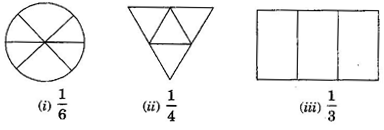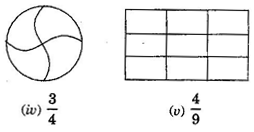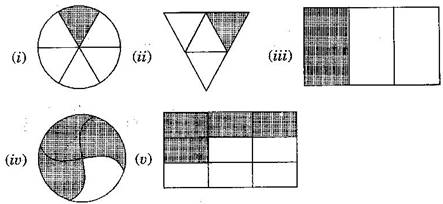NCERT Solutions for Class 6 Maths Exercise 7.1

###### Question 3.Identify the error, if any?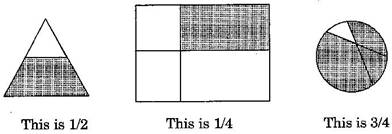All the figures are not equally divided. For making fractions, it is necessary that figure is to be divided in equal parts.

###### Question 4.What fraction of a day is 8 hours?

Since, 1 day = 24 hours.

Therefore, the fraction of 8 hours = {tex}\frac{8}{{24}} = \frac{1}{3}{/tex}

NCERT Solutions for Class 6 Maths Exercise 7.1

###### Question 5.What fraction of an hour is 40 minutes?

Since, 1 hour = 60 minutes.

Therefore, the fraction of 40 minutes = {tex}\frac{{40}}{{60}} = \frac{2}{3}{/tex}

###### Question 6.Arya, Abhimanyu and Vivek shared lunch. Arya has brought two sandwiches, one made of vegetable and one of jam. The other two boys forgot to bring their lunch. Arya agreed to share his sandwiches so that each person will have an equal share of each sandwich.

(a)How can Arya divide his sandwiches so that each person has an equal share?

(b)What part of a sandwich will each boy receive?

(a) Arya will divide each sandwich into three equal parts and give one part of each sandwich to

each one of them.

(b) {tex}1 \times \frac{1}{3} = \frac{1}{3}{/tex}

NCERT Solutions for Class 6 Maths Exercise 7.1

###### Question 7.Kanchan dyes dresses. She had to dye 30 dresses. She has so far finished 20 dresses. What fraction of dresses has she finished?

Total number of dresses = 30

Work finished = 20

Fraction of finished work = {tex}\frac{{20}}{{30}} = \frac{2}{3}{/tex}

###### Question 8.Write the natural numbers from 2 to 12. What fraction of them are prime numbers?

Natural numbers from 2 to 12: 2, 3, 4, 5, 6, 7, 8, 9, 10, 11, 12

Prime numbers from 2 to 12: 2, 3, 5, 7, 11

Hence, fraction of prime numbers = {tex}\frac{5}{{11}}{/tex}

NCERT Solutions for Class 6 Maths Exercise 7.1

###### Question 9.Write the natural numbers from 102 to 113. What fraction of them are prime numbers?

Natural numbers from 102 to 113: 102, 103, 104, 105, 106, 107, 108, 109, 110, 111, 112, 113

Prime numbers from 102 to 113: 103, 107, 109, 113

Hence fraction of prime numbers = {tex}\frac{4}{{12}} = \frac{1}{3}{/tex}

###### Question 10.What fraction of these circles have ‘X’s in them?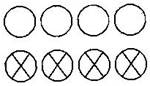Total number of circles = 8 and number of circles having ‘X’ = 4

Hence, the fraction = {tex}\frac{4}{8}{/tex}

NCERT Solutions for Class 6 Maths Exercise 7.1

###### Question 11.Kristin received a CD player for her birthday. She bought 3 CDs and received 5 others as gifts. What fraction of her total CDs did she buy and what fraction did she receive as gifts?

Total number of CDs = 3 + 5 = 8

Number of CDs purchased = 3

Fraction of CDs purchased = {tex}\frac{3}{8}{/tex}

## NCERT Solutions for Class 6 Maths Exercise 7.1

NCERT Solutions Class 6 Maths PDF (Download) Free from myCBSEguide app and myCBSEguide website. Ncert solution class 6 Maths includes text book solutions from Class 6 Maths Book . NCERT Solutions for CBSE Class 6 Maths have total 14 chapters. 6 Maths NCERT Solutions in PDF for free Download on our website. Ncert Maths class 6 solutions PDF and Maths ncert class 6 PDF solutions with latest modifications and as per the latest CBSE syllabus are only available in myCBSEguide.

## CBSE app for Students

To download NCERT Solutions for Class 6 Maths, Social Science Computer Science, Home Science, Hindi English, Maths Science do check myCBSEguide app or website. myCBSEguide provides sample papers with solution, test papers for chapter-wise practice, NCERT solutions, NCERT Exemplar solutions, quick revision notes for ready reference, CBSE guess papers and CBSE important question papers. Sample Paper all are made available through the best app for CBSE students and myCBSEguide website.Test Generator

Create question paper PDF and online tests with your own name & logo in minutes.myCBSEguide

Question Bank, Mock Tests, Exam Papers, NCERT Solutions, Sample Papers, Notes

### 2 thoughts on “NCERT Solutions for Class 6 Maths Exercise 7.1”

1. Thanks for giving me such a great thanks for this

2. very good,very usefull for children to practice ,I would like to request for more questions…..